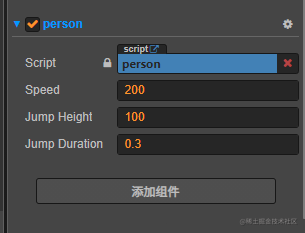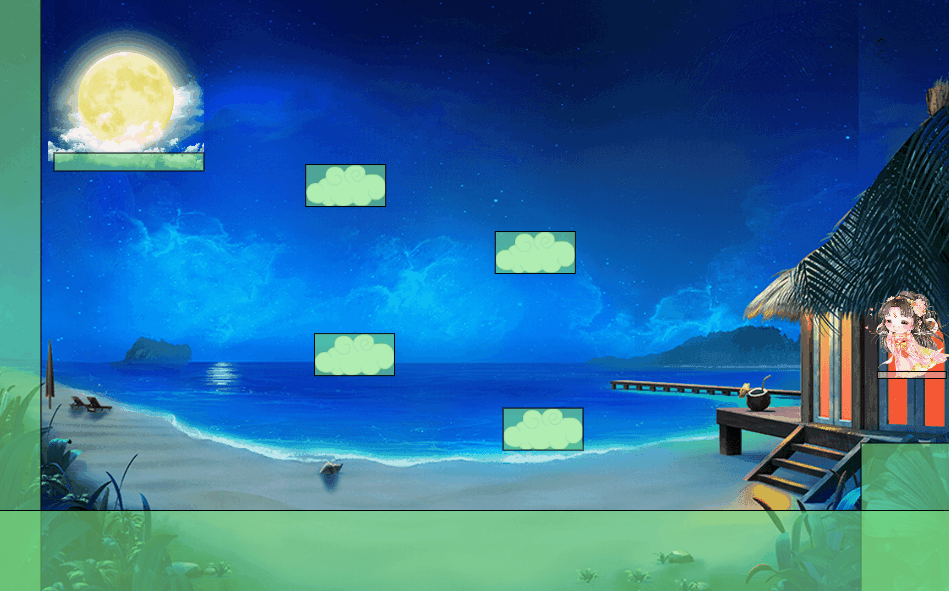# 用Cocos Creator让嫦娥一步步跳上月亮# 中秋## Cocos Creator

1. Cocos Creator免费
2. 容易上手，使用`JavaScript`或者`Typescript`
3. 中文开发文档
4. 与Unity类似的界面，同样是数据驱动的开发模式

## 静态布局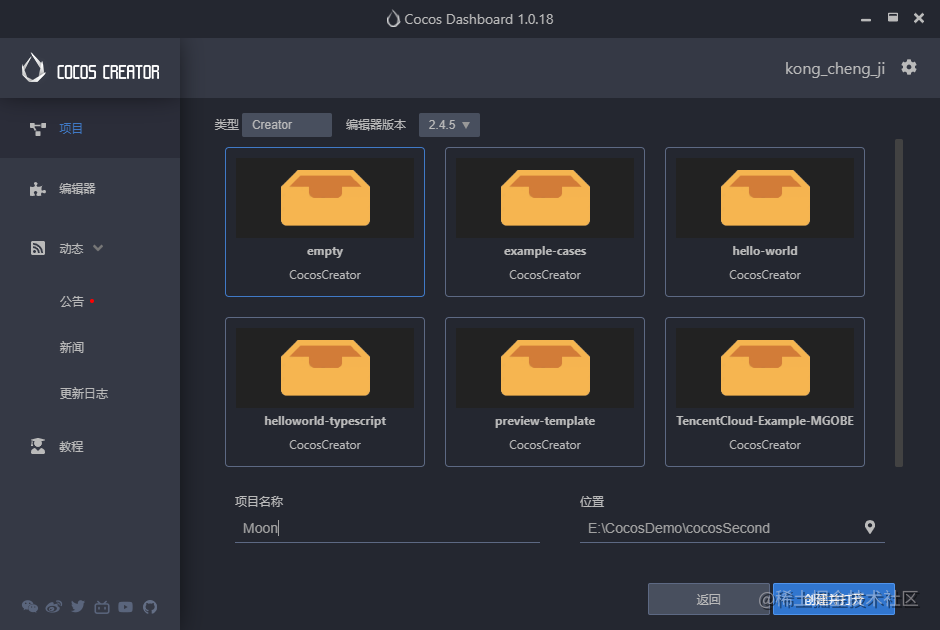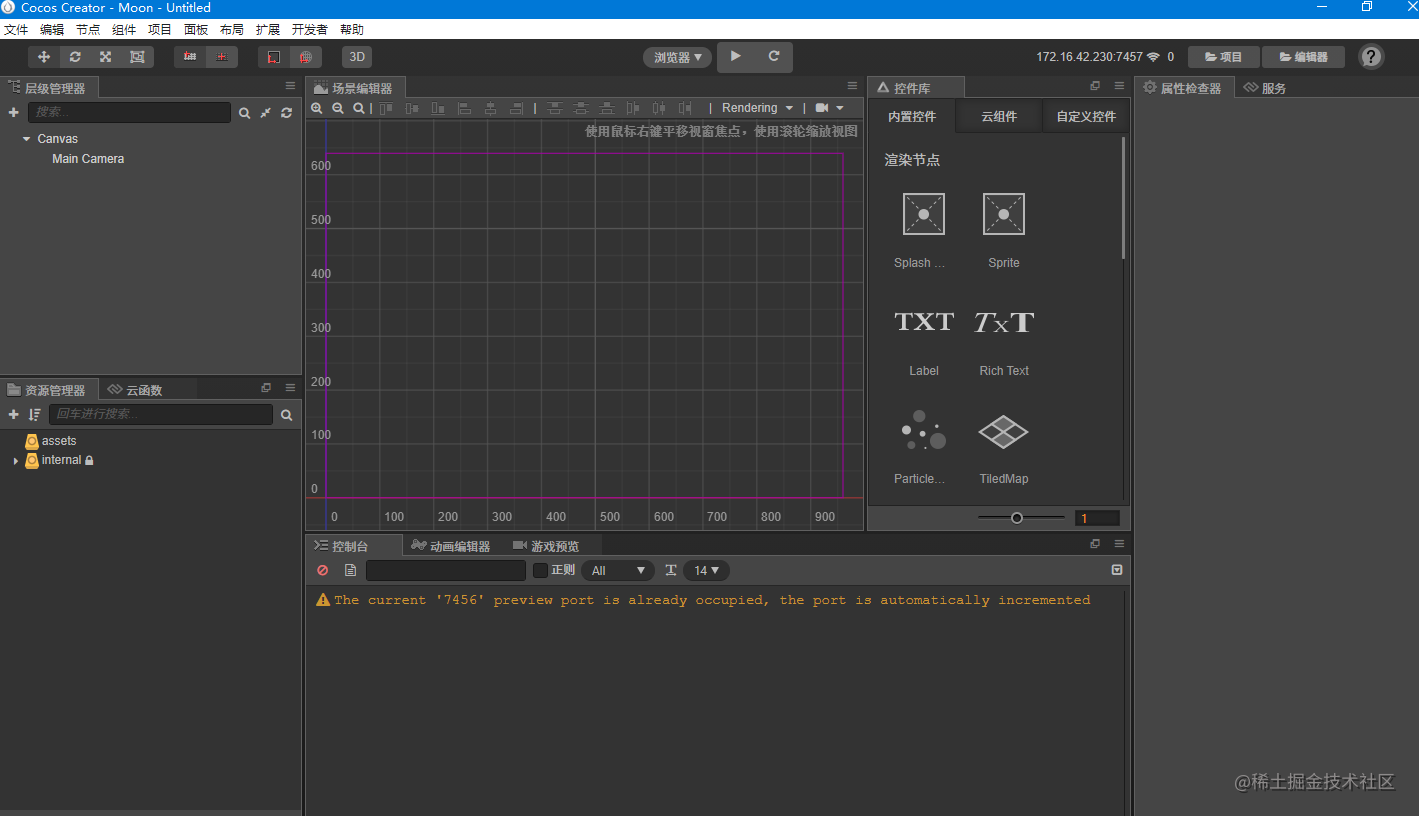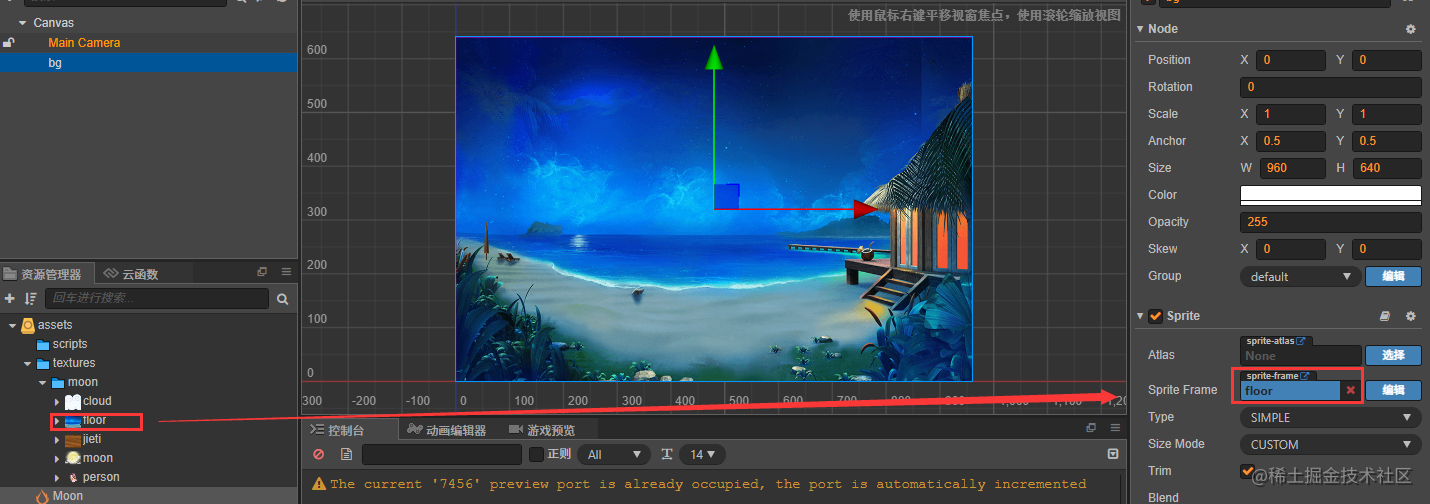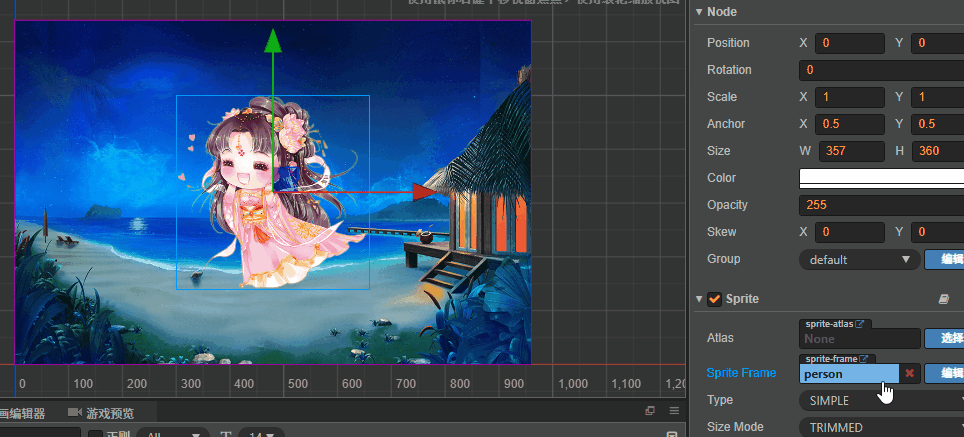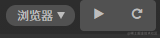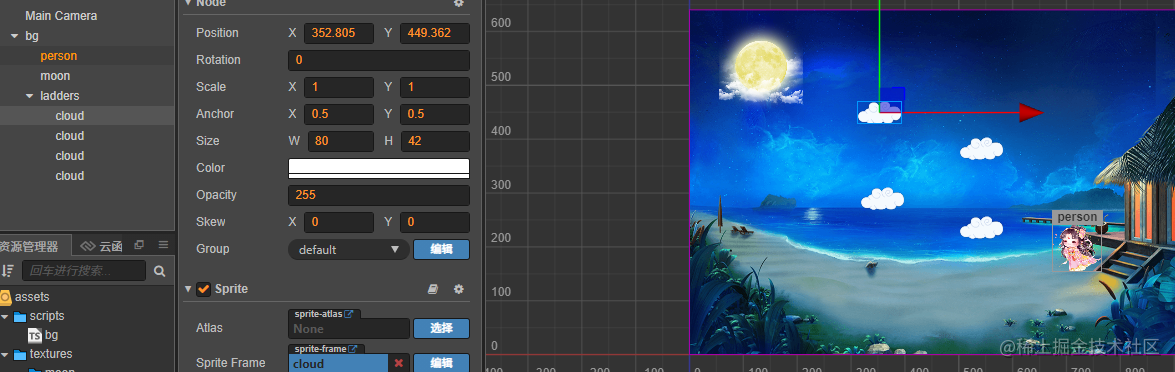## 添加物理系统

`onLoad()`中添加：`cc.director.getPhysicsManager().enabled = true`，这句话能添加物理管理器。然后把`bg.ts`拖动到`bg节点`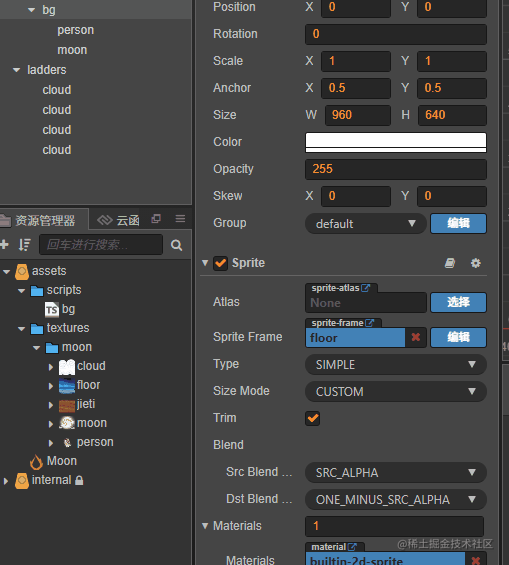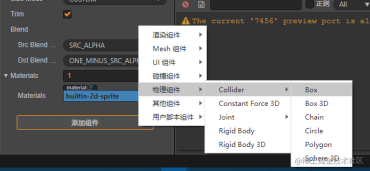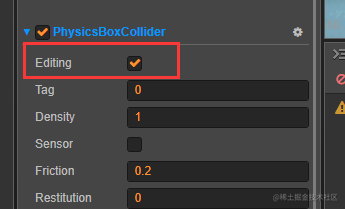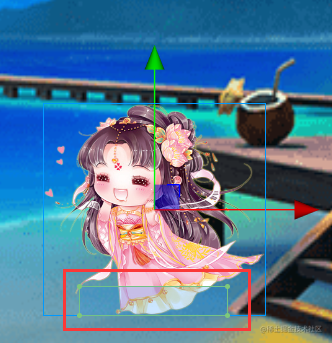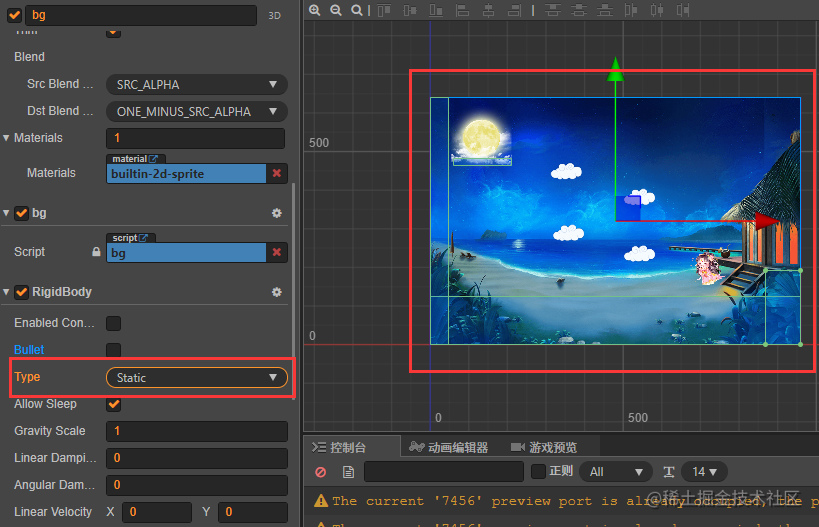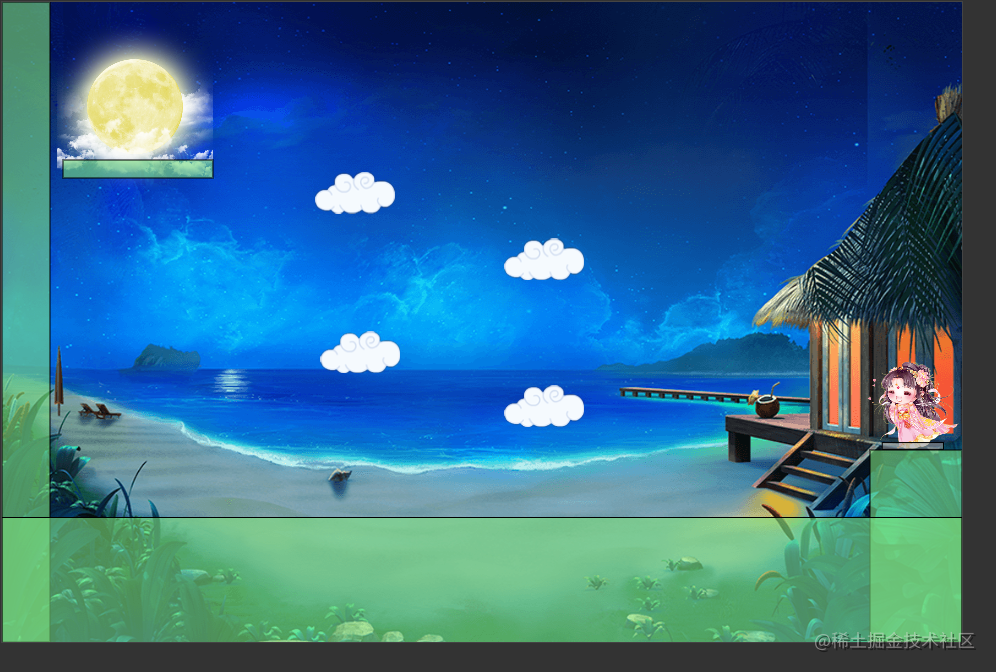## 运动脚本

``````cc.systemEvent.on(cc.SystemEvent.EventType.KEY_DOWN, this.onKeyDown, this);
cc.systemEvent.on(cc.SystemEvent.EventType.KEY_UP, this.onKeyUp, this);

``````cc.macro.KEY.a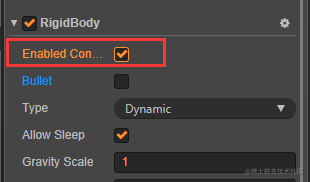Person.ts代码：

``````@ccclass
export default class Person extends cc.Component {
// 人物速度
@property(cc.Integer)
speed:number = 0;
// 人物方向
sp:cc.Vec2 = cc.v2(0, 0);
lv:cc.Vec2 = cc.v2(0, 0);
private Input:{} = { };

@property
jumpHeight:number =  0;
// 主角跳跃持续时间
@property
jumpDuration:number = 0;
// 是否正在跳跃
isJumping:boolean = false;

// LIFE-CYCLE CALLBACKS:
// 初始化键盘输入监听
cc.systemEvent.on(cc.SystemEvent.EventType.KEY_DOWN, this.onKeyDown, this);
cc.systemEvent.on(cc.SystemEvent.EventType.KEY_UP, this.onKeyUp, this);
}

onKeyDown(event: cc.Event.EventKeyboard) {
this.Input[event.keyCode] = 1;
}
onKeyUp(event: cc.Event.EventKeyboard) {
this.Input[event.keyCode] = 0;
}

moveState() {
let anim:string;
let scaleX = Math.abs(this.node.scaleX);
if (this.Input[cc.macro.KEY.a] || this.Input[cc.macro.KEY.left]) {
this.sp.x = -1;
this.node.scaleX = scaleX;
} else if (this.Input[cc.macro.KEY.d] || this.Input[cc.macro.KEY.right]) {
this.sp.x = 1;
this.node.scaleX = -scaleX;
} else {
this.sp.x = 0;

}
if (this.Input[cc.macro.KEY.w] || this.Input[cc.macro.KEY.up]) {
this.sp.y = -1;
} else {
this.sp.y = 0;
}

if (this.sp.x != 0) {
this.lv.x = this.sp.x * this.speed;
} else {
this.lv.x = 0
}

if (this.sp.y != 0) {
if (this.isJumping) return;
cc.tween(this.node).then(this.runJumpAction()).start();
}
}

// 跳跃方法
runJumpAction():cc.Tween {
this.isJumping = true;
// 跳跃上升
let jumpUp = cc.tween().by(this.jumpDuration, {y: this.jumpHeight}, {easing: 'sineOut'})
return jumpUp;
}
onBeginContact(contact:any, selfCollider, otherCollider) {
this.isJumping = false;
}
update (dt) {
this.lv = this.node.getComponent(cc.RigidBody).linearVelocity;
this.moveState();
this.node.getComponent(cc.RigidBody).linearVelocity = this.lv;
}
}